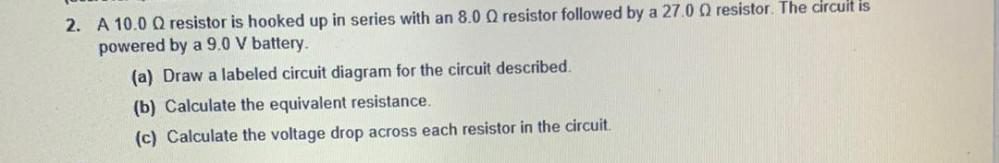Question:

# 2. A 10.00 resistor is hooked up in series with an 8.00 resistor followed by a 27.0 resistor. The circuit is powered by a 9.0 V2. A 10.00 resistor is hooked up in series with an 8.00 resistor followed by a 27.0 resistor. The circuit is powered by a 9.0 V battery. (a) Draw a labeled circuit diagram for the circuit described (b) Calculate the equivalent resistance. (c) Calculate the voltage drop across each resistor in the circuit.